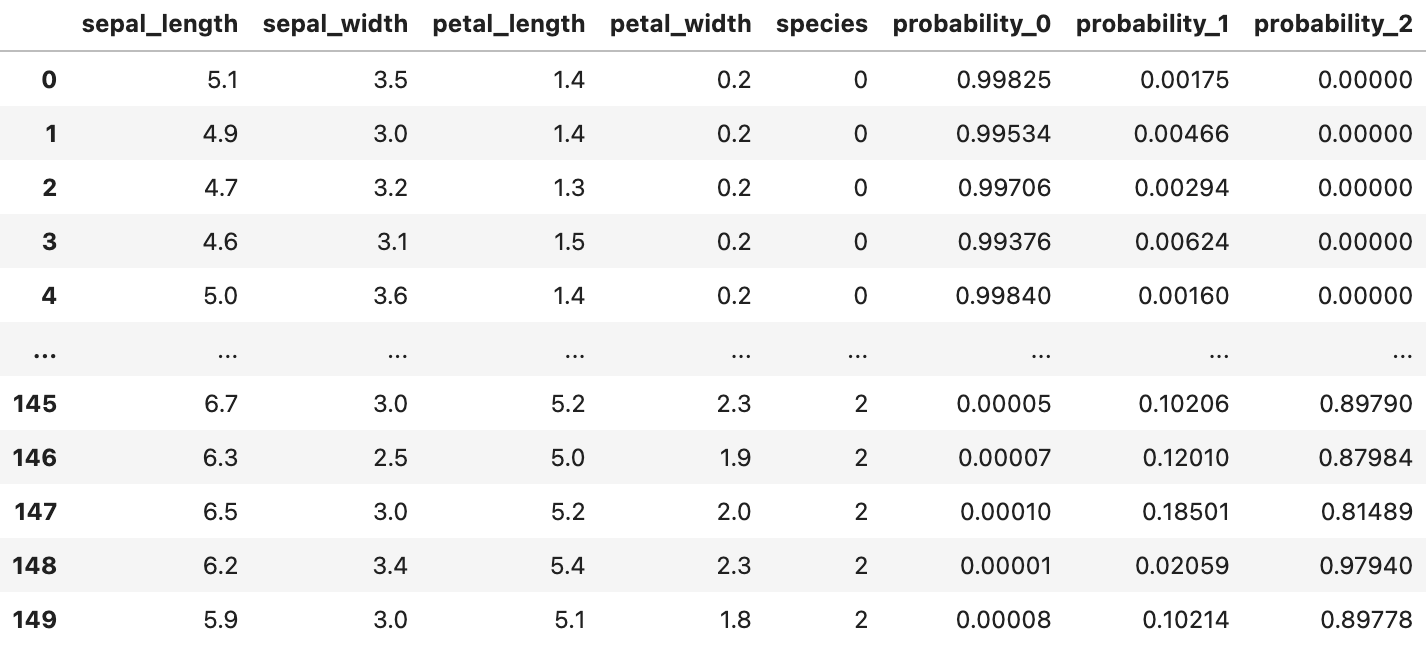# Multiclass Classification

## Registering a Multiclass Classification Model

Suppose you would like to register a multiclass classification model for the following dataset.Following is an example of how you would construct a `fdl.ModelInfo` object and register such a model.

### 📘categorical_target_class_details

For multiclass models, the `categorical_target_class_details` argument is required.

This argument should be a list of your target classes in the order that your model outputs predictions for them.

``````PROJECT_ID = 'example_project'
DATASET_ID = 'iris_data'
MODEL_ID = 'multiclass_model'

dataset_info = client.get_dataset_info(
project_id=PROJECT_ID,
dataset_id=DATASET_ID
)

model_target = 'species'
model_outputs = [
'probability_0',
'probability_1',
'probability_2'
]
model_features = [
'sepal_length',
'sepal_width',
'petal_length',
'petal_width'
]

model_info = fdl.ModelInfo.from_dataset_info(
dataset_info=dataset_info,
dataset_id=DATASET_ID,
target=model_target,
outputs=model_outputs,